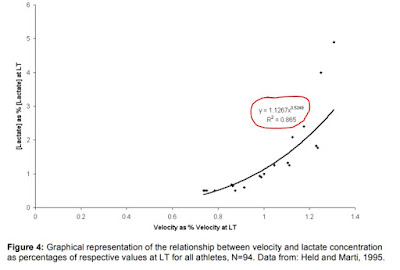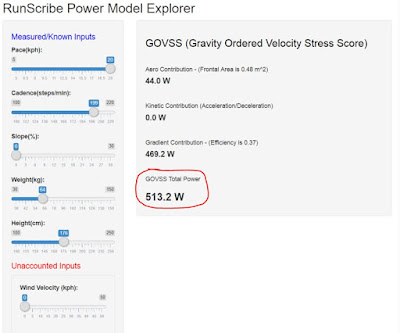## Saturday, November 11, 2017

### Technical Review of the Runscibe GOVSS Running Power Model

I would like to discuss the Gravity Ordered Velocity Stress Score (GOVSS) model for running, provide some comments in blue italics and simultaneously compare to Coggan metrics such as NP, IF and TSS (all trademarked under Training Peaks).

Tim Clark at Runscribe told me their RS+ will now incorporate the Skiba GOVSS model. Being open in what they are implementing is greatly appreciated.

Just off the bat : Dr. Andrew Coggan recently commented on the Stryd Forum that many of his metrics and guidelines developed for cycling don't 'necessarily' apply to running, nor should one consider TSS, rTSS, sTSS, BikeScore, GOVSS, RSS etc as completely interchangeable.

That said, here's the GOVSS algorithm as proposed by Phil Skiba, 2006 :

1. Find the athlete’s velocity at LT by a 10 km to one hour maximal run.

Note : Presumably the thinking behind this is that a true 10K intensity is the maximum intensity in the intensity continuum where a delicate homeostatic balance in physiological parameters is maintained. Research has also shown that speed or power at LT is a valid predictor of endurance CYCLING performance (r = 0.88 for cycling, Coyle. el al 1991). In cycling, the previous statement has been debated because cycling is so much more than a single discipline of TT'ing. Running on the other hand is predominantly a time trial against the clock so applying a LT limited power model may not be so unreasonable. This is probably also the basis for Stryd's CP and RSS paradigm.

2. Convert this LT limited velocity to a LT limited power value using Equation 7. "Lactate limited power" may also be called "lactate adjusted power".

Note : The equation converts a "threshold" velocity to a "threshold" power using Prof. di Prampero's power-balanced supply-demand equation for running energetics which expresses the metabolic RATE of running in terms of COST of energy C. The equation is then modified into a power by multilying with a speed specific efficiency. The efficiency that is used in power equation can be rated to different speeds with a simple linear equation based on the finding that efficiency varies linearly 0.5-0.7 at 8.33 m/s (30 km/h) in a reasonably linear fashion (Cavangna and Kaneko 1976, Arsac 2001).  A 5th order regression model from Minetti (2002) is used to apply a general running surface to the cost for better acounting for slope effects.

3. Analyze the data from a particular workout from an athlete’s log, computing 120 second rolling averages from velocity and slope data.

Note - Before applying rolling averages, the following equations are applied to figure out instantaneous GOVSS based power. Equations are from the reference down in the bottom of this article.Fig 1 a,b,c : Series of equations used to convert energy cost of running to lactate adjusted power
Referring to Fig 1a, Caero, the energy cost of overcoming air resistance = k.n‑1.d2.t2, and k is the constant of air friction (in kg-1. m-1 ) with n = 0.5. Ckin, the energy cost of acceleration = 0.5.n-1.d t-2 , with n = 0.25.  It is important to note that equation was minimally "modified" to suit events ranging from 800m to 5K. But the original form was successfully used to predict performances for middle and long distance running.

Referring to Fig 1b, the equation describes the velocity independant energy cost C to cover any distance. In the absense of a slope, this defaults to 3.6 - 4.2 J·kg-1·m-1.  In the presence of a slope i, it becomes a 5th order regression equation.

Referring to Fig 1c, the equation converts metabolic rate to a mechanical power to weight ratio available for locomotion by multiplying metabolic cost with the separate efficiencies. This becomes a total power cost to run as it includes both the cost of things like COM motion and limb swing.

All values of cost C (kinetic, air resistance and slope related) are calculated as rolling averages over 120 seconds. Skiba wrote that this was to account for the fact that the original 5th order cost of running model was validated to the 800m.

4. Raise the values in step 3 to the 4th power.

Note - Skiba investigated LT dynamics in relation to running speed in a group of running subjects and applied a simple power fit (as Coggan did with his data). The regression fit said that the lactate levels in the body were a function of the speed of running raised to the power of 3.5. The power exponent was 4.2 in the top 10% of the subjects and 2.5 in the bottom 10%.  A power exponent of 3.5 became a middle ground to apply to the entire population of tested subjects (N = 94). Presumably, the 3.5 has got rounded up to 4 by Skiba to make it easy to apply but I question this. Why not just stick to the original exponent?Fig 2 : The basis behind an exponent in the power model (Point 4).

5. Average values from step 4.

Note - Same algorithm as Coggan's Normalized Power.

6. Take the 4th root of step 5. This is the Lactate-Normalized Power.

Note : The general idea behind normalizing is that a normalized power is an ESTIMATED power output that an athlete can maintain for the same physiological cost if the power output had been perfectly constant. Even though the approach wrt Coggan's NP calculation remains similar, where the difference lies is in that whereas NP s a 30 second rolling average, LT NP for running is a 120 second rolling average.  In cycling, 30 seconds was found to be a response time for many physiological variables but some have come out and contradicted the usefulness of NP. I won't go into that.

7. Divide Lactate Normalized Power by Threshold Power from step 2 to get the Intensity Weighting Fraction.

Note : IWF is similar to the IF concept.

8. Multiply the Lactate Normalized Power by the duration of the workout in seconds to obtain the normalized work performed in joules.

Note : Key idea that can be lost on people here is that this is a normalized work in KJ and it is a TOTAL amount of work because the power equation in Step 2 used a metabolic efficiency to convert metabolic rate to power.

9. Multiply value obtained in step 8 by the Intensity Weighting Fraction to get a raw training stress value.

Note : The resulting training stress, is by virtue of the math, expressed in work KJ . This may not relate to a TSS implementation of KJ because of the difference in mathematics involved (see above points).

10. Divide the values from step 9 by the amount of work performed during the 10k to 1 hr test (threshold power in watts x number of seconds).

Note : This step is basically again normalizing the amount of "normalized work" from the workout file to the amount of work from the LT test.

11. Multiply the number from step 10 by 100 to obtain the final training stress in GOVSS.

Note :  The Coggan TSS is graded based on a similar idea that a 1 hour ride at FTP corresponds to 100 TSS. Therefore, GOVSS also becomes relative to the score of 100.

Hopefully the details in the algorithm show in what respects the GOVSS is different relative to  a cycling based TSS.

Implementation Examples

Below is an example of GOVSS calculated power for a runner performing intervals at 20 kph and running at about 199 SPM on a slope of 0%. The model uses a calculated frontal area of 0.48sq.m to estimate the aero contribution for power. You can play around with this power model here.Fig 3 : Estimated GOVSS power to run at steady state at 20 kph on flat ground. Runner weight = 64 kg. Assumed wind = 0 kph.

Below is a GOVSS PMC from my running data implemented in Golden Cheetah. This is just to show you an example.Fig 4 : Example of a GOVSS implementation in Golden Cheetah. GC's Triscore PMC uses GOVSS for runs. However, the GOVSS is possibly based on pace, rather than power. This needs confirming with Mark Liveradge.

Concluding Remarks

1. The GOVSS model takes into account the energy cost of running and how that varies as a function of running gradient, acceleration and wind resistance. For example, even in slow to medium speed running regimes as those in endurance running, the energy cost to tackle wind resistance is alone atleast 8%.

2. The GOVSS model gives the total energy expenditure of running per km and therefore, includes the effects of internal power needed to swing the arms and legs relative to center of mass (see Physics of Running Power). Therefore, a GOVSS based power may end up being higher than a purely external power that does not account for these aspects.

3. GOVSS relies heavily on measured speed and gradient. Errors in measurement propagate to the calculated GOVSS power.

4. It still has be known whether the originators of some of the equations behind GOVSS intended to have it be applied to distances ranging from 3K all the way to the marathon. This point needs investigation and testing.

5. GOVSS for running and TSS for cycling use different mathematics and philosophy. TSS from cycling applied for running will be a mis-application, as implied by Andrew Coggan.

6. As with TSS, the GOVSS scoring scheme relies on base data from a sample population of runners who were tested under controlled settings in a laboratory. The statistical power of such fits and accompanying simplications are not always high Application of scoring metrics to a general population of athletes who are not tested in the laboratory come with the acceptance of a risk of deviation.

7. Scoring workouts to a curve based on 100 brings it's own debate.

For example, the popular notion of an FTP as corresponding to the maximum power that can be applied to a bike in approximately 1 hour has been challenged by Dr. Coggan himself several times. It is somewhat of an urban legend, popular but untrue.

The “approximately one hour” component of the definition has since been clarified to range between 30-75 minutes, depending on the individual.

Similarly, the CP reported by Stryd Powercenter is said to reflect a sustainable duration of about 50 minutes as per Stryd.

If the TTE in a cycling and running situation are different numbers and if this varies from individual to individual, one could then question the use of the value of 100 in the grading as that corresponding to a 1 hour duration.

In the absence of better alternatives to apply in an athlete's Performance Management chart, the GOVSS, TSS, RSS etc all can be used purely for their modeling value but practitioners must refrain from using them interchangeably.

Reference

Calculation of Power Output and Quantification of Training Stress in Distance Runners: The Development of the GOVSS Algorithm (Skiba, 2006) : Link